# Colleges with the lowest SAT scores in Utah

Top 6 colleges in Utah with the lowest SAT scores
Looking for the colleges with the lowest SAT scores in Utah? Well you're in luck! We've compiled a national college database and have created a list of the top 6 universities with the lowest SAT scores in Utah below. If you are not a good test taker or worried about your test scores, this list is for you. These are the schools whose applicants had the lowest average SAT scores in Utah, which means that you can get into these colleges with a lower SAT score. We also include each college's ACT scores and acceptance rate so that you can see where you would have the easiest time getting in. Read on to find out more.

## Dixie State University SAT scores

The average SAT score for Dixie State University is 1000.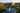The average SAT score of 1000 breaks down into:

• SAT math: 500

The average ACT score for Dixie State University is 19 and their acceptance rate is 100%.

## Weber State University SAT scores

The average SAT score for Weber State University is 1080.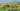The average SAT score of 1080 breaks down into:

• SAT math: 540

The average ACT score for Weber State University is 21 and their acceptance rate is 100%.

## Southern Utah University SAT scores

The average SAT score for Southern Utah University is 1117.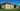The average SAT score of 1117 breaks down into:

• SAT math: 550

The average ACT score for Southern Utah University is 23 and their acceptance rate is 75.8%.

## Utah State University SAT scores

The average SAT score for Utah State University is 1170.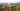The average SAT score of 1170 breaks down into:

• SAT math: 580

The average ACT score for Utah State University is 24 and their acceptance rate is 89.1%.

## University of Utah SAT scores

The average SAT score for University of Utah is 1262.The average SAT score of 1262 breaks down into:

• SAT math: 635

The average ACT score for University of Utah is 26 and their acceptance rate is 62.1%.

## Brigham Young University SAT scores

The average SAT score for Brigham Young University is 1315.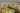The average SAT score of 1315 breaks down into:

• SAT math: 655